# HBSE 10th Class Maths Solutions Chapter 5 Arithmetic Progressions Ex 5.3

Haryana State Board HBSE 10th Class Maths Solutions Chapter 5 Arithmetic Progressions Ex 5.3 Textbook Exercise Questions and Answers.

## Haryana Board 10th Class Maths Solutions Chapter 5 Arithmetic Progressions Ex 5.3

Question 1.
Find the sum of the following APs :
(i) 2, 7, 12, ………….., to 10 terms.
(ii) – 37, – 33, – 29, ………….., to 12 terms.
(iii) 0.6, 1.7, 2.8, …………., to 100 terms.
(iv) $$\frac{1}{15}, \frac{1}{12}, \frac{1}{10}, \ldots$$ to 11 terms.
Solution :
(i) The given sequence of AP is : 2, 7, 12, …………….., to 10 terms
Here, a = 2
d = a2 – a1
=7 – 2 = 5 and n = 10
We know that
Sn = $$\frac{n}{2}$$ [2a + (n – 1)d]
⇒ S10 = $$\frac{10}{2}$$ [2 × 2 + (10 – 1) × 5]
⇒ S10 = 5[4 + 9 × 5]
⇒ S10 = 5 [4 + 45]
⇒ S10 = 5 × 49 = 245
Hence, the sum of 10 terms of given AP = 245.(ii) The given sequence of AP is : – 37, – 33, – 29, …………., to 12 terms
Here, a = – 37
d = a2 – a1
= – 33 – (- 37)
= – 33 + 37 = 4
and n = 12
We know that
Sn = $$\frac{n}{2}$$ [2a + (n – 1)d]
⇒ S12 = $$\frac{n}{2}$$ [2 × (- 37) + (12 – 1) × 4]
⇒ S12 = 6[- 74 + 11 × 4]
⇒ S12 = 6[- 74 + 44]
⇒ S12 = 6 × (- 30) = – 180
Hence, the sum of 12 terms of given AP = – 180.

(iii) The given sequence of AP is : 0.6, 1.7, 2.8, …………., to 100 terms.
Here, a = 0.6
d = a2 – a1
= 1.7 – 0.6 = 1.1 and n = 100
We know that
Sn = $$\frac{n}{2}$$ [2a + (n – l)d]
⇒ S100 = $$\frac{100}{2}$$ [2 × 0.6 + (100 – 1) × 1.1]
⇒ S100 = 50 [1.2 + 99 × 1.1]
⇒ S100 = 50[1.2 + 108.9]
⇒ S100 = 50 × 110.1 = 5505
Hence, the sum of 100 terms of given AP = 5505.(iv) The given sequence of AP is : $$\frac{1}{15}, \frac{1}{12}, \frac{1}{10}, \ldots$$ to 11 terms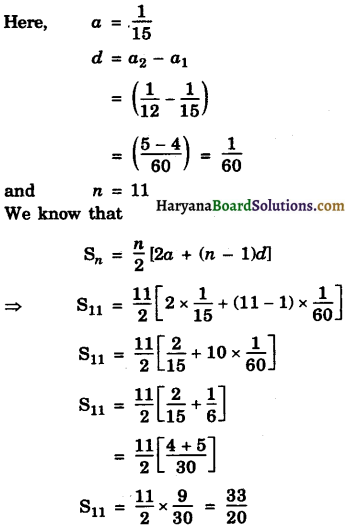Hence, the sum of 11 terms of given AP = $$\frac{33}{20}$$.Question 2.
Find the sum of the following APs given below :
(i) 7 + 10$$\frac{1}{2}$$ + 14 + …………. + 84
(ii) 34 + 32 + 30 + …………. + 10
(iii) – 5 + (- 8) + (- 11) + …………. + (- 230)
Solution:
(i)Wehave, 7 + 10$$\frac{1}{2}$$ + 14 + …………. + 84
It is an AP.
Here, a = 7
d = a2 – a1
d = 10$$\frac{1}{2}$$ – 1
d = $$\frac{21-14}{2}$$ = $$\frac{7}{2}$$
and l = an = 84
⇒ a + (n – 1)d = 84
⇒ 7 + (n – 1) × $$\frac{7}{2}$$ = 84
$$\frac{7}{2}$$ n – $$\frac{7}{2}$$ = 84 – 7
$$\frac{7}{2}$$ n – $$\frac{7}{2}$$ = 77
$$\frac{7}{2}$$ n = 77 + $$\frac{7}{2}$$
$$\frac{7}{2}$$ n = $$\frac{154+77}{2}$$
⇒ n = $$\frac{161}{7}$$ = 23
We know that
Sn = $$\frac{n}{2}$$ [a + l]
Sn = $$\frac{23}{2}$$ [7 + 84]
S23 = $$\frac{23}{2}$$ × 91
S23 = 1046$$\frac{1}{2}$$
Hence, S23 = 1046$$\frac{1}{2}$$(ii) We have, 34 + 32 + 30 + …………….. + 10.
It is an AP.
Here, a = 34
d = a2 – a1
= 32 – 34 = – 2 and
l = an = 10
⇒ a + ( n – 1 )d = 10
⇒ 34 + (n – 1) × (- 2) = 10
⇒ – 2n + 2 = 10 – 34
⇒ – 2n + 2 = – 24
⇒ – 2n = – 24 – 2
⇒ – 2n = – 26
⇒ n = $$\frac{-26}{-2}$$ = 13
We know that
Sn = $$\frac{n}{2}$$ [a + l]
S13 = $$\frac{13}{2}$$ [34 + 10]
= $$\frac{13}{2}$$ × 44
S13 = 286
Hence S13 = 286.

(ill) Wehave, – 5 + (- 8) + (- 11) + …………… + (- 230)
It is an AP.
Here, a = – 5
d = a2 – a1
= (- 8) – (- 5)
and l = an = – 230
⇒ a + (n – 1) d = – 230
⇒ – 5 + (n – 1) × (- 3) = – 230
⇒ – 3n + 3 = – 230 + 5
⇒ – 3n + 3 = – 225
⇒ – 3n = – 225 – 3
⇒ – 3n = – 228
⇒ n = $$\frac{-228}{-3}$$ = 76
We know that Sn = $$\frac{n}{2}$$ [a + l]
S76 = $$\frac{76}{2}$$ [- 5 – 230] = 38 × (- 235)
S76 = – 8930
Hence, S76 = – 8930.Question 3.
In an AP :
(i) given a = 5, d = 3, an = 50, find n and Sn.
(ii) given a = 7, a13 = 35, find d and S13.
(iii) given a12 = 37, d = 3, find a and S12.
(iv) given a3 = 15, S10 = 125, find d and a10.
(v) given d = 5, S9 = 75, find a and a9.
(vi) given a = 2, d = 8, Sn = 90, find n and an.
(vii) given a = 8, an = 62, Sn = 210, find n and d.
(viii) given an = 4, d = 2, Sn = – 14, find n and a.
(ix) given a = 3, n = 8, Sn = 192, find d.
(x) given l = 28, Sn = 144, and there are total 9 terms. Find a.
Solution :
(i) We have, a = 5, d = 3, an = 50
We know that
a + (n – l)d = an
⇒ 5 + (n – 1) × 3 = 50
⇒ 5 + 3n – 3 = 50
⇒ 2 + 3n – 50
⇒ 3n = 50 – 2 = 48
⇒ n = $$\frac{48}{3}$$ = 16
We know that
Sn = $$\frac{n}{2}$$ [2a + (n – 1)d]
S16 = $$\frac{16}{2}$$ [2 × 5 + (16 – 1) × 3]
S16 = 8 [10 + 15 × 3]
= 8 [10 + 45]
S16 = 8 × 55 = 440
Hence, n = 16, S16 = 440.(ii) We have, a = 7, a13 = 35
We know that
a + (n – 1) d = an
7 + (13 – 1) d = 35
7 + 12d = 35
12d = 35 – 7 = 28
d = $$\frac{28}{12}=\frac{7}{3}$$
We know that
Sn = $$\frac{n}{2}$$ [2a + (n – 1)d]
[ l = a13 = 35]
S13 = $$\frac{13}{2}$$ [7 + 35]
= $$\frac{13}{2}$$ × 42 = 273
Hence d = $$\frac{7}{3}$$, S13 = 273.

(iii) We have, d = 3, a12 = 37
We know that
a + (n – 1 )d = an
⇒ o + (12 – 1) × 3 = 37
⇒ a + 11 × 3 = 37
⇒ a + 33 = 37
⇒ a = 37 – 33 = 4
We know that
Sn = $$\frac{n}{2}$$ [a + l]
S12 = $$\frac{12}{2}$$ [4 + 37] = 6 × 41
[∵ l = a12 = 37]
S12 = 246.
Hence, a = 4, a12 = 246.(iv) We have, a3 = 15, S10 = 126
Now, a3 – 15
a + (3 – 1) d = 15
[∵ an = a + (n – 1)d]
a + 2d = 15 ……………(1)
and S10 = 125
$$\frac{n}{2}$$ [2a + (10- 1)d] = 125
[∵ Sn = $$\frac{n}{2}$$ (2a +(n – 1)d)]
5 [2a + 9d] = 125
2a + 9d = $$\frac{125}{5}$$
2a + 9d = 25 ………………(2)
Multiplying equation (1) by 2 and subtracting (2) from it, we get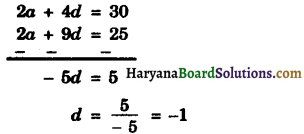Putting the value of d in equation (1), we get
a + 2 × (- 1) = 16
a – 2 = 15
a = 15 + 2
a = 17
Now, a10 = 17 + (10 – 1) × (- 1)
a10 = 17 + 9 × (- 1)
a10 = 17 – 9
a10 = 8
Hence, d = – 1 and a10 = 8.

(v) We have, d = 5, S9 = 75
⇒ $$\frac{9}{2}$$ [2a + (9 – 1) × 5] = 75
[∵ Sn = $$\frac{n}{2}$$ [2a + (n – 1)d]]
⇒ $$\frac{9}{2}$$ [2a + 8 × 5] = 75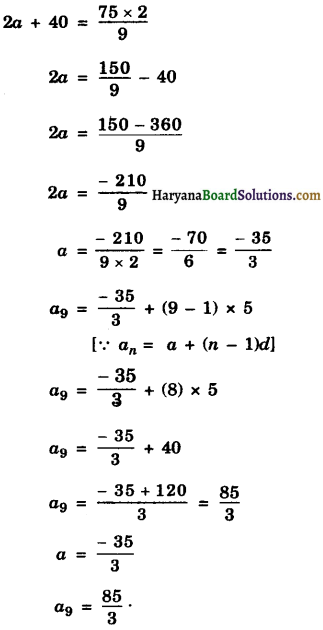(vi) We have, a = 2, d = 8, Sn = 90
⇒ $$\frac{n}{2}$$ [2a + (n – 1) d] = 90
[∵ Sn = $$\frac{n}{2}$$ [2a + (n – 1)d]]
$$\frac{n}{2}$$ [2 × 2 + (n – 1)d] = 90
[∵ Sn = $$\frac{n}{2}$$ [2a + (n – 1)d]]
⇒ $$\frac{n}{2}$$ [2 × 2 + (n – 1) × 8] =90
⇒ $$\frac{n}{2}$$ [4 + 8n – 8] = 90
⇒ $$\frac{n}{2}$$ [8n – 4] = 90 × 2
⇒ 8n2 – 4n = 180
⇒ 2n2 – n = 45
⇒ 2n2 – n – 45 = 0
⇒ 2n2 – (10 – 9)n – 45 = 0
⇒ 2n2 – 10n + 9n – 45 =0
⇒ 2n(n – 5) + 9(n – 5) = 0
[∵ 2 × – 45 = – 90
10 × – 9 = – 90
10 – 9 = 1]
⇒ (n – 5) (2n + 9) = 0
n – 5 = 0 or 2n + 9 = 0
n = 5 or n = – $$\frac{9}{2}$$
Reject n = – $$\frac{9}{2}$$ [∵ n ≠ – $$\frac{9}{2}$$]
So, n = 5
Now, an = a + (n – 1)d
⇒ a5 = 2 + (5 – 1) × 8
⇒ a5 = 2 + 4 × 8
⇒ a5 = 2 + 32
⇒ a5 = 34
Hence, n = 5 and a5 = 34.

(vii) We have, a = 8, an = 62, Sn = 210
Now, Sn = 210
$$\frac{9}{2}$$ [a + l] = 210
$$\frac{9}{2}$$ [8 + 62] = 210 [∵ l = an = 62]
$$\frac{n}{2}$$ × 70 = 210
35n = 210
n = $$\frac{210}{35}$$ = 6
Now, an = 62
⇒ 8 + (6 – 1) d = 62
⇒ [an = a + (n – 1)d]
⇒ 8 + 5d = 62
⇒ 5d = 62 – 8
⇒ 5d = 54
⇒ d = – $$\frac{54}{5}$$
Hence, n = 6, d = $$\frac{54}{5}$$.(viii) We have, d = 2, Sn = – 14, an = 4
⇒ a + (n – 1) × 2 = 4[∵ an = a + (n – 1) d]
⇒ a + 2n – 2 = 4
⇒ a + 2n = 4 + 2
⇒ a + 2n = 6
⇒ a = 6 – 2n ……………….(i)
Now, Sn = – 14
⇒ $$\frac{n}{2}$$ [a + l] = – 14
⇒ n[6 – 2n + 4] = – 14 × 2
[From (1) Put a = 6 – 2n]
⇒ n [10 – 2n] = – 28 [∵ l = an = 4]
⇒ 10n – 2n2 = – 28
⇒ 2n2 – 10n – 28 = 0
⇒ n2 – 5n – 14 = 0
⇒ n2 – (7 – 2)n – 14 = 0
⇒ n2 – 7n + 2n – 14 = 0
[∵ 1 × – 14 = – 14
7 × – 2 = – 14
7 – 2 = 5]
⇒ n(n – 7) + 2(n – 7) = 0
⇒ (n – 7)(n + 2) = 0
⇒ n – 7 = 0 or n + 2 = 0
⇒ n = 7 or n = – 2
Reject n = – 2 [∵ n ≠ – 2]
So, n = 7
Substituting the value of n in equation (1), we
a = 6 – 2 × 7
⇒ a = 6 – 14
⇒ a = – 8.
Hence, n = 7, a = – 8.(ix) We have, a = 3, n = 8, Sn = 192
i.e. S8 = 192
⇒ $$\frac{8}{2}$$ [2 × 3 + (8 – 1) d] = 192
[∵ Sn = $$\frac{n}{2}$$ (2a + (n – 1) d)]
⇒ 4 [6 + 7d] = 192
⇒ 6 + 7d = 48
⇒ 7d = 48 – 6
⇒ 7d = 42
⇒ d = $$\frac{42}{7}$$ = 6
Hence, d = 6.

(x) We have, n = 9, l = 28, Sn = 144
$$\frac{n}{2}$$ [a + l] = 144
$$\frac{9}{2}$$ [a + 28] = 144
a + 28 = $$\frac{144 \times 2}{9}$$
a + 28 = 32
a = 32 – 28 = 4
Hence, a = 4.Question 4.
How many terms of the AP: 9, 17, 25, ………. must be taken to give a sum of 636?
Solution:
The given sequence of AP is: 9, 17, 25, ………….
Here, a = 9
d = a2 – a1
= 17 – 9 = 8
and Sn = 636 (Given)
$$\frac{n}{2}$$ [2a + (n – 1)d] = 636
$$\frac{n}{2}$$ [2 × 9 + (n – 1) × 8] = 636
n [18 + 8n – 8] = 636 × 2
n [10 + 8n] = 1272
10n + 8n2 = 1272
8n2 + 10n – 1272 = 0
4n2 + 5n – 636 = 0
4n2 + (53 – 48)n – 636 = 0
[∵ – 636 × 4 = – 2544
53 × – 48 = – 2544
53 – 48 = 5]
4n2 + 53n – 48n – 636 = 0
n(4n + 53) – 12(4n + 53) = 0
(4n + 53)(n – 12) = 0
4n + 53 = 0 or n – 12 = 0
n = – $$\frac{53}{4}$$ or n = 12
Reject, n = – $$\frac{53}{4}$$
[∵ n cannot be fraction]
So, n = 12.
Hence, 12 terms of the given AP must be taken to give a sum of 636.

Question 5.
The first term of an AP is 5, the last term 1845 and the sum is 400. Find the number of terms and the common difference.
Solution :
We have, a = 5, l = an = 45, Sn = 400
$$\frac{n}{2}$$ [a + l] = 400
$$\frac{n}{2}$$ [15 + 45] = 400
n × 50 = 400 × 2
50n = 800
n = $$\frac{800}{50}$$
n = 16
Now, an = 45
a + (n – 1) d = 45
5 + (16 – 1)d = 45
5 + 15d = 45
15d = 45 – 5
15d = 40
d = $$\frac{40}{15}$$
d = $$\frac{8}{3}$$
Hence, n = 16, d = $$\frac{8}{3}$$.Question 6.
The first and last terms of an AP are 17 and 350 respectively. 1f the common difference is 9, how many terms are there and what is their sum?
Solution:
We have, a = 17, d = 9, l = an = 350
a + (n – 1)d = 350
17 + (n – 1)9 = 350
17 + 9n – 9 = 350
8 + 9n = 350
9n = 350 -8
9n = 342
n = $$\frac{342}{9}$$ = 38
Now, Sn = $$\frac{n}{2}$$ [a + l]
S38 = $$\frac{38}{2}$$ [17 + 350]
S38 = 19 × 367
S38 = 6973
Hence, the number of terms = 38
and their sum = 6973.

Question 7.
Find the sum of first 22 terms of an AP in which d = 7 and 22nd term is 149.
Solution :
We have, d = 7, n = 22, a22 = 149
a + (22 – 1) × 7 = 149
[∵ an = a + (n – 1)d]
a + 21 × 7 = 149
a + 147 = 149
a = 149 – 147
a = 2
Now, Sn = $$\frac{n}{2}$$ [a + l]
S22 = $$\frac{22}{2}$$ [2 + 149]
[∵ l = an = 149]
S22 = 11 × 151
S22 = 1661
Hence, S22 = 1661.Question 8.
Find the sum of first 51 terms of an AP whose second and third terms are 14 and 18 respectively.
Solution :
We have, n = 51, a2 = 14, a3 = 18
d = a2 – a1
⇒ d = 18 – 14
d = 4
Therefore, a3 = a – d
= 14 – 4 = 10
Sn = $$\frac{n}{2}$$ [2a + (n – 1)d]
S51 = $$\frac{51}{2}$$ [2 × 10 + (51 – 1) × 4]
S51 = $$\frac{51}{2}$$ [20 + 50 × 4]
⇒ S51 = $$\frac{51}{2}$$ [20 + 200]
⇒ S51 = $$\frac{51}{2}$$ 
⇒ S51 = 51 × 110
⇒ S51 = 5610
Hence, S51 = 5610.

Question 9.
If the sum of first 7 terms of an AP is 49 and that of 17 terms is 289. Find the sum of first n terms.
Solution :
We have, S7 = 49
⇒ $$\frac{7}{2}$$ [2a + (7 – 1)5] = 49
[∵ Sn = $$\frac{n}{2}$$ [2a + (n – 1)d]]
2a + 6d = $$\frac{49 \times 2}{7}$$
2a + 6d = 14
a + 35 = 7 ……………..(1)
S17 = 289
$$\frac{17}{2}$$ [2a + (17 – 1) 5] = 289
2a + 16d = $$\frac{289 \times 2}{17}$$
2a + 16d = 34
a + 8d = 17
Subtracting equation (2) from equation (1), we get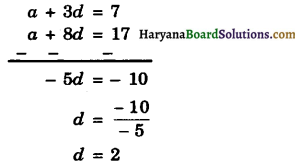Putting the value of 5 in equation (1), we get
a + 3 × 2 = 7
⇒ a + 6 = 7
⇒ a = 7 – 6
⇒ a = 1
Now, Sn = $$\frac{n}{2}$$ [2a + (n – 1)d]
⇒ Sn = $$\frac{n}{2}$$ [2 x 1 + (n – 1) x 2]
⇒ Sn = $$\frac{n}{2}$$ [2 + 2n – 2]
⇒ Sn = $$\frac{n}{2}$$ [2n]
⇒ Sn = n2
Hence Sn = n2.Question 10.
Show that a1, a2, …………., an form an AP, where an is defined as below :
(i) an = 3 + 4n
(ii) an = 9 – 5n.
Also find the sum of the first 15 terms in each case.
Solution :
(i) We have, an = 3 + 4n
Putting n = 1, 2, 3, 4,…………. in this equation, we get
a1 = 3 + 4 × 1
⇒ a1 = 7
a2 = 3 + 4 × 2
⇒ a2 = 3 + 8
⇒ a = 11
a3 = 3 + 4 × 3
⇒ a3 = 3 + 12
a3 = 15
a4 = 3 + 4 × 4
⇒ a4 = 3 + 16
⇒ a4 = 19 …………… and so on.
Therefore, the sequence is : 7, 11, 15, 19, …………..
a2 – a1 = 11 – 7 = 4
a3 – a2 = 15 – 11 = 4
a4 – a3 = 19 – 15 = 4
∵ a2 – a1 = a3 – a2 = a4 – a3
The given sequence forms an AP Proved.
Here a = 7, d = a2 – a1 = 11 – 7 = 4, n = 15
Now, Sn = $$\frac{n}{2}$$ [2a + (n – 1)d]
⇒ S15 = $$\frac{15}{2}$$ [2 × 7 + (15 – 1)
⇒ S15 = $$\frac{15}{2}$$ [14 + 14 × 4]
⇒ S15 = $$\frac{15}{2}$$ [14 + 56]
⇒ S15 = $$\frac{15}{2}$$ × 70
S15 = 15 × 35
S15 = 525
Hence, S15 = 525.

(ii) We have, an = 9 – 5n
Putting n = 1, 2, 3, 4, …………. in this equation,
a1 = 9 – 5 × 1
a1 = 9 – 5
⇒ a1 = 4
a2 = 9 – 5 × 2
⇒ a2 = 9 – 10
⇒ a2 = -1
a3 = 9 – 5 × 3
⇒ a3 = 9 – 15
a3 = – 6
a4 = 9 – 5 × 4
⇒ a4 = 9 – 20
⇒ a4 = – 11
Therefore, the sequence is : 4, – 1, – 6, – 11,…………..
a2 – a1 = -1 – 4 = – 5
a3 – a2 = – 6 – (- 1)
= – 6 + 1 = – 5
a4 – a3 = – 11 – (- 6)
= – 11 + 6 = – 5
∵ a2 – a1 = a3 – a2 = a4 – a3
∴ The given sequence forms an AP. Proved
Here, a = 4, d = a2 – a2 = – 1 – 4 = – 5, n = 15
Now, Sn = $$\frac{n}{2}$$ [2a + (n – 1)d]
⇒ S15 = $$\frac{15}{2}$$ [2 × 4 + (15 – 1) (- 15)]
⇒ S15 = $$\frac{15}{2}$$ [8 + 14 × (- 5)]
⇒ S15 = $$\frac{15}{2}$$ [8 – 70]
⇒ S15 = $$\frac{15}{2}$$ × (- 62)
⇒ S15 = 15 × (- 31)
⇒ S15 = – 465
Hence, S15 = – 465.Question 11.
If the sum of the first n terms of an AP is 4n – n2, what is the first term (i.e., S4)? What is the sum of first two terms? What is the second term? Similarly, find the 3rd, the 10th and 71th terms.
Solution :
We have, Sn = 4n – n2
Putting n = 1, We get
S1 = 4 × 1 – (1)2
⇒ S1 = 4 – 1
⇒ S1 = 3
⇒ a1 = 3
Putting n = 2, we get
S2 = 4 × 2 – (2)2
⇒ S2 = 8 – 4
⇒ S2 = 4
⇒ a4 + a2 = 4
⇒ 3 + a2 = 4
⇒ a2 = 4 – 3
⇒ a2 = 1
Now, d = a2 – a1
= 1 – 3 = – 2
a3 = 3 + (3 – 1) × (- 2)
[∵ an = a + (n – 1)d]
a3 = 3 + 2 × (- 2)
a3 = 3 – 4
a3 = – 1
a10 = 3 + (10 – 1) × (- 2)
a10 = 3 + 9 × (- 2)
a10 = 3 – 18 = -15
And an = 3 + (n – 1) × (- 2)
an = 3 – 2n + 2
an = 5 – 2n
Hence a1 = 3, S2 = 4, a2 = 1, a3 = – 1, a10 = – 15, an = 56 – 2n.

Question 12.
Find the sum of the first 40 positive integers divisible by 6.
Solution:
The first 40 positive integers divisible by 6 are : 6, 12, 18, 24, …, 240.
Since, each number is divisible by 6.
So it is an AP in which
a = 6,
d = a2 – a1
= 12 – 6 = 6,
n = 40 and l = 240
Now, S40 = [16 + 240]
[∵ Sn = (a + l)]
S40 = 20 × 246
S40 = 4920
Hence, the sum of the first 40 positive integers divisible by 6 = 4920.Question 13.
Find the sum of the first 15 multiples of 8.
Solution :
The first 15 multiples of 8 are 8, 16, 24, 32, …, 120.
Since each number is multiple of 8, So, it is an AP in which
a = 8,d = a2 – a1 = 16 – 8 = 8, n = 5 and l = 120
Now, S15 – a1 = $$\frac{15}{2}$$ [8 + 120]
[∵ Sn = $$\frac{n}{2}$$ (a + l)]
S15 = $$\frac{15}{2}$$ × 128
S15 = 15 × 64
S15 = 960
Hence, the sum of the first 15 multiples of 8 = 960.

Question 14.
Find the sum of the odd numbers between 0 and 50.
Solution :
The odd numbers between 0 and 50 are : 1, 3, 5, 7, ………….., 49.
Since, difference between each two consecutive odd numbers is 2. So it is an AP.
In which a = 1, d = a2 – a1 = 3 – 1 = 2, l = an =49
a + (n – 1)d = 49[∵ a + (n – 1) d = an]
1 + (n – 1) × 2 = 49
1 + 2n – 2 = 49
2n – 1 = 49
2n = 49 + 1
2n = 50
n = $$\frac{50}{2}$$
n = 25
Now, Sn = $$\frac{n}{2}$$ [a + l]
S25 = $$\frac{25}{2}$$ [1 + 49]
S25 = $$\frac{25}{2}$$ × 50
S25 = 25 × 25
S25 = 625
Hence, the sum of odd numbers between 0 and 50 = 625.Question 15.
A contract on construction job specifies a penalty for delay of completion beyond a certain date as follows: ₹ 200 for the first day, ₹ 250 for the second day, ₹ 300 for the third day, etc …, the penalty for each succeeding day being ₹ 50 more than for the preceding day. How much money the contractor has to pay as penalty, if he has delayed the work by 30 days?
Solution :
We have,
Penalty for the first day = ₹ 200
Penalty for the second day = ₹ 250
Penalty for the third day = ₹ 300
So, the sequence of penalty is :200, 250, 300, …………..
Here, a = 200
The penalty for each succeeding day is ₹ 50
more than for each the preceding day.
So, d = 250 – 200 = 50
and n = 30
Now, S30 = $$\frac{30}{2}$$ [2 × 200 + (30 – 1) x50]
[∵ Sn = $$\frac{n}{2}$$ [2a + (n – 1)d]
S30 = 15[400 + 29 × 50]
S30 = 15 [400 + 1450]
S30 =15 × 1850
S30 = 27750
Hence, the penalty paid for 30 days = ₹ 27750.

Question 16.
A sum of ₹ 700 is to be used to give seven cash prizes to students of a school for their overall academic performance. If each prize is ₹ 20 less than its preceding prize, find the value of each of the prizes.
Solution :
Let Ist Prize be ₹ a
Then 2nd prize = ₹ (a – 20)
3rd prize = ₹ (a – 20 – 20) = (a – 40)
Thus, the seven prize are a,
(a – 20), (a – 40), …………….., (a – 120)
Since, each prize is Rs. 20 less than its pre ceding prize. So it is an AP.
Here first term (a) = a
Common difference (d)
= a2 – a1
= a – 20 – a = – 20
Number of prizes (n)= 7 and
an = l = a – 120
Sum of prizes (Sn) = 700
Now, S7 = 700
⇒ l [a + a – 120] = 700
[∵ Sn = $$\frac{n}{2}$$ [a + l]
[2a – 120] = $$\frac{700 \times 2}{7}$$
⇒ 2a – 120 = 200
⇒ 2a = 200 + 120 = 320
⇒ a = $$\frac{320}{2}$$
⇒ a = 160
So, given prizes are 160, 160 – 20, 160 – 40, 160 – 60, 160 – 80, 160 – 100, 160 – 120,
i.e. 160, 140, 120, 100, 80, 60, 40.
Hence, seven prizes are ₹ 160, ₹ 140, ₹ 120, ₹ 100, ₹ 80, ₹ 60, ₹ 40.Question 17.
In a school, students thought of planting trees in and around the school to reduce air pollution. It was decided that the number of trees, that each section of each class will plant, will be the same as the class, in which they are studying, e.g. a section of class I will plant 1 tree, a section of class II will plant 2 trees and so on till class XII. There are three sections of each class. How many trees will be planted by the students?
Solution :
Trees planted by three sections of class I = 3 × 1 = 3
Trees planted by three sections of Class II = 3 × 2 = 6
Trees planted by three sections of Class III = 3× 3 = 9
Trees planted by three sections of class IV = 3 × 4 = 12
Trees planted by three section of class XII = 3 × 12 = 36
. .
. .
. .
. .
. .
∴ The sequence of trees is : 3, 6, 9, 12, …, 36
a2 – a1 = 6 – 3 = 3
a3 – a2 = 9 – 6 = 3
a4 – a3 = 1293
∵ a2 – a1 = a3 – a2 = a4 – a3
The given sequence of trees forms an AP.
Here a = 3, d = a2 – a1 = 6 – 3 = 3,
an = l = 36, n = 12
Total number of trees will be planted = Sn
= $$\frac{n}{2}$$ [a + l]
= $$\frac{12}{2}$$ [3 + 36]
= 6 × 39 = 234
Hence, the total number of trees will be planted = 234.Question 18.
A spiral is made up of succes¬sive semicircles, with centres alternately at A and B, starting with centre at A, of radii 0.5 cm, 1.0 cm, 1.5 cm, 2.0 cm … as shown in figure. What is the total length of such a spiral made up of thirteen consecutive semicircles? (Take π = $$\frac{22}{7}$$)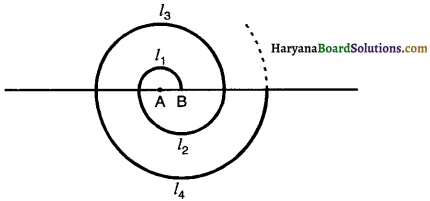Solution:
Let l1, l2, l3, l4, ……………….., l13 be the lengths (Circumferences) of semicircles of radii r1 = 0.5 cm, r2 = 1.0 cm, r3 = 1.5 cm, r4 = 2.0 cm,… respectively.
Then
l1 = πr1
= π × 0.5
= $$\frac{\pi}{2} \mathrm{~cm}=\frac{22}{14} \mathrm{~cm}=\frac{11}{7}$$ cm

l2 = πr2
= π × 1.0
= π cm = $$\frac{22}{7}$$ cm

l3 = πr3
= π × 1.5
= 1.5π cm = 1.5 × $$\frac{22}{7}$$ = $$\frac{33}{7}$$ cm

l4 = πr4
= π × 2.0
= 2π cm = $$\frac{44}{7}$$ cm
. .
. .
. .
. .
. .
l13 = πr13
= π × 6.5
= 6.5π = 6.5 × $$\frac{22}{7}$$
= $$\frac{143}{7}$$
The sequence of length of spiral is $$\frac{11}{7}, \frac{22}{7}, \frac{33}{7}, \frac{44}{7}, \ldots, \frac{143}{7}$$.
a2 – a1 = $$\frac{22}{7}-\frac{11}{7}=\frac{11}{7}$$
a3 – a2 = $$\frac{33}{7}-\frac{22}{7}=\frac{11}{7}$$
a4 – a3 = $$\frac{44}{7}-\frac{33}{7}=\frac{11}{7}$$
∵ a2 – a1 = a3 – a2 = a4 – a3 =
The sequence of length of spiral forms an
Here a = $$\frac{11}{7}$$
d = a2 – a1
= $$\frac{22}{7}-\frac{11}{7}=\frac{11}{7}$$
l = $$\frac{143}{7}$$,
and n = 13
Now, the length of such a spiral made up of thirteen consecutive semicircles = S13
S13 = $$\frac{13}{2}\left[\frac{11}{7}+\frac{143}{7}\right]$$
[∵ Sn = $$\frac{n}{2}$$ [a + l]]
⇒ S13 = $$\frac{13}{2}\left[\frac{154}{7}\right]$$
⇒ S13 = $$\frac{13}{2}$$ × 22
⇒ S13 = 13 × 11
⇒ S13 = 143.
Hence, the length of a spiral made up of thir¬teen consecutive semicircles = 143 cm.Question 19.
200 logs are stacked in the following manner : 20 logs in the bottom row, 19 in the next row, 18 in the row next to it and so on (see in figure). In how many rows are the 200 logs placed and how many logs are in the top row?Solution :
Sequence of logs row-wise is as follows :
20, 19, 18, 17, ………………
a2 – a1 = 19 – 20 = – 1
a3 – a2 = 18 – 19 = – 1
a4 – a3 = 17 – 18 = – 1
∵ a2 – a1 = a3 – a2 = a4 – a3
∴ Sequence of logs row-wise forms an AP.
Here, a = 20, d = a2 – a1 = 19 – 20 = – 1, Sn = 200
$$\frac{n}{2}$$ [2a + (n – 1)d] = 200
n[2 × 20 + (n – 1) × (- 1)] = 200 × 2
n[40 – n + 1] = 400
n [41 – n] = 400
41n – n2 = 400
n2 – 41n + 400 = 0
n2 – (25 + 16)n + 400 = 0
[1 × 400 = 400
25 × 16 = 400
25 + 16 = 41]
n2 – 25n – 16n + 400 = 0
⇒ n(n – 25) – 16(n – 25) = 0
⇒(n – 25) (n – 16) = 0
⇒ n – 25 = 0 or n – 16 = 0
⇒ n = 25 or n = 16
If n = 25
Number of logs in top row = a25
= 20 + (25 – 1) × (- 1) [∵ an = a + (n – 1)d]
= 20 – 24 = – 4
– 4 is not meaningful
So, we reject n = 25.
∴ n = 16
∴ Number of logs in top row = a16
= 20 + (16 – 1) × (- 1)
= 20 – 15 = 5
Hence, the number of rows = 16 and
the number of logs in top row = 5.Question 20.
In a potato race, a bucket is placed at the starting point, which is 5 m from the first potato and the other potatoes are placed 3 m apart in a straight line. There are ten potatoes in the line (see figure).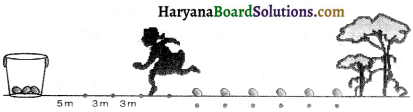A competitor starts from the bucket, picks up the nearest potato, runs back with it, drops it in the bucket, runs back to pick up the next potato, runs to the bucket to drop it in, and she continues in the same way until all the potatoes are draped in the bucket. What is the total distance the competitor has to run ?
Solution :
Distance run to pick up the 1st potato = 2 × 5 = 10 m
Distance run to pick up the 2nd potato = 2 × (5 + 3) = 16 m
Distance run to pick up the 3rd potato = 2 × (5 + 3 + 3) = 22 m
Distance run to pick up the 4th potato = 2 × (5 + 3 + 3 + 3) = 28 m
The sequence of distances is 10, 16, 22, 28.
a2 – a1 = 16 – 10 = 6
a3 – a2 – 22 – 16 = 6
a4 – a3 = 28 – 22 = 6
∵ a2 – a1 = a3 – a2 = a4 – a3
The sequence forms an AP.
Here, a = 10, d = a2 – a1 = 16 – 10 = 6, n = 10
Now, total distance run by a competitor = S10
= $$\frac{10}{2}$$ [2 × 10 + (10 – 1) × 61]
[∵ Sn = $$\frac{n}{2}$$ [2a + (n – 1)d)]]
= 5 [20 + 9 × 6]
= 5 [20 + 54]
= 5 = 370 m
Hence, the total distance run by competitor = 370 m.